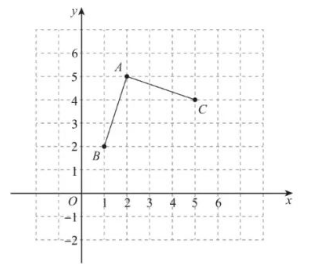(1) $\angle B A C=$
(2) 若点 $D$ 为整点, 且满足 $\triangle A B D \cong \triangle A C D$, 直接写出点 $D$ 的坐标 (写出两个即可).(1) 90
(2) $(1,7),(3,3),(4,1),(5,-1)$ 等中的两个均可.
①点击 首页查看更多试卷和试题 , 点击查看 本题所在试卷